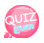# Variable declaration

I always thought I had to study JavaScript, but I had postponed it. (LAZY!! -_-) However, this time, I had to make a bulletin board while I was working. The PHP part went smoothly, but I had difficulty because I did not know JavaScript well. I need to sanction the error on the bulletin board for user's writing, but I needed JavaScript for this function. So I started to study JavaScript. For example, when trying to type an emai addressl, special characters must be entered as @ and. people may enter other special characters such as !! \$\$ (# \$ (#) #). You need a function that prevents you from typing those wrong type of special characters. Javascript is needed in these cases.

Anyway, let's learn about Variables! ^-^

### What is a variable?

It can be definded as a space for storing a certain value. So let's say a value of 20, for example. The value is 20, and a space for store 20 is a variable. Then how could we make that space or variable? Just type. If you want to call a space a, you can write a. You can write any values if following some rules. So if you want a, write a. And what if you would like to save 20 in a ? Use "=", which creates and stores a space a, and also creates a value of 20. That is,

`a = 20;`

If you write like this, the variable a has a value of 20. If you enter 30, you will have 30. Please enter a value and enter ;, which means the end of a tag. If the value you want to enter is a number, then you could just write down the number. On the other hand, if you would like to enter letters as the value, then just wrap it it quato as below.

`a = "Hello.";`

Let's check how the output will be when using document.write();

### JavaScript

```num = 20;
str = "Hello.";
document.write(num);
document.write("<br />");
document.write(str);```

If you look at the source above, a variable named num is declared, being given a value of 20. And a variable named str is declared , being given a value of Hello. We also add the tag, Document.write ("<br />"); to make it easier to see. Tags can be used wrapped in "". So let's type in the entire source.

```<!DOCTYPE html>
<html>
<meta charset="utf-8" />
<title>JavaScript</title>
<script>
num = 20;
str = "Hello.";
document.write(num);
document.write("<br />");
document.write(str);
</script>
<body>
</body>
</html>```

### Calculation using variables

Now let's do arithmetic operations using variables. For example, substitute 5 for a and 10 for b, and add a and b to c. Since it's not easy undertand, let's look at the source.

A = 5; Assign 5 to a
b = 10; Assign 10 to b
That is, the value of a is 5, the value of b is 10, and the value of a + b is 15.
Therefore, c has a value of 15, which is the sum of a and b.
Document.write (c); Outputs the value of c.

Try running the source.

```<!DOCTYPE html>
<html>
<meta charset="utf-8" />
<title>JavaScript</title>
<script>
a = 5;
b = 10;
c = a + b;
document.write(c);
</script>
<body>
</body>
</html>```

Type in the above source and check the results.

The screen will show the result,15. Using the above source, let's create a source that will solve the 4-arithmetic values.

#### the remaindet %

the remaninder is like; 5 divided by 3, then the remainder is 2.

```<!DOCTYPE html>
<html>
<meta charset="utf-8" />
<title>JavaScript</title>
<script>
a = 5;
b = 10;
sub = a - b;
mul = a * b;
div = b / a;
mod = b % a;
document.write("<br />");
document.write("subtract"+sub);
document.write("<br />");
document.write("multiply"+mul);
document.write("<br />");
document.write("divide"+div);
document.write("<br />");
document.write("rest"+mod);
</script>